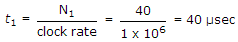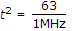# Electronics and Communication Engineering - Digital Electronics

16.

The number of switching functions of 3 variables are

 A. 3 B. 8 C. 16 D. 32

Explanation:

23 = 8.

17.

The applications of shift registers are

1. Time delay
2. Ring counter
3. Serial to parallel data conversion
4. Serial to serial data conversion
Which of the above are correct?

 A. 1, 2, 3 B. 2, 3, 4 C. 1, 2, 3, 4 D. 1, 2, 4

Explanation:

Shift registers are used for all these applications.

18.

Assertion (A): ECL is fast as compared to TTL.

Reason (R): ECL dissipates less power than TTL.

 A. Both A and R are correct and R is correct explanation of A B. Both A and R are correct but R is not correct explanation of A C. A is true, R is false D. A is false, R is true

Explanation:

ECL dissipates more power than TTL.

19.

Assertion (A): Synchronous counter has higher speed of operation than ripple counter

Reason (R): Synchronous counter uses high speed flip flops.

 A. Both A and R are correct and R is correct explanation of A B. Both A and R are correct but R is not correct explanation of A C. A is true, R is false D. A is false, R is true

Explanation:

Higher speed is due-to the reason that clock pulses in synchronous counter are applied simultaneously to all the flip-flops.

20.

What will be the maximum conversion time in 6 bit dual slope A/D converter uses a reference of -6v and a 1 MHz clock. It uses a fixed count of 40 (101000).

 A. 108 sec B. 63 μ sec C. 40 sec D. 80 μ sec

Explanation:for t2 maximum all bits should be 111111 = (63)10Maximum conversion time = 63 + 40 = 103 μ sec and it takes 5 μ sec to transfer N2 to the O/P register.

tcmax = 108 μ sec.